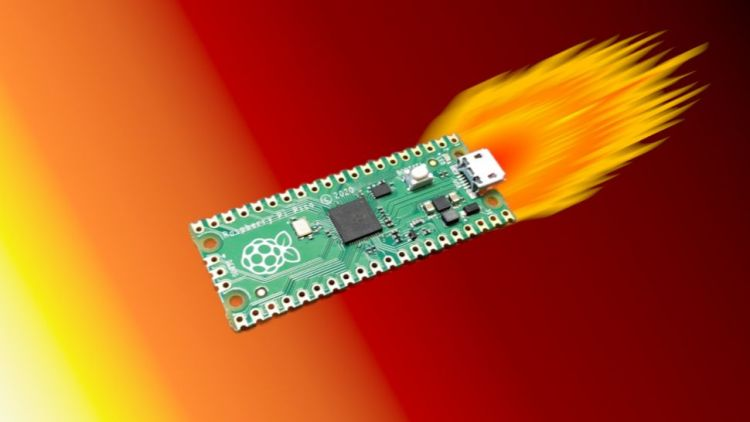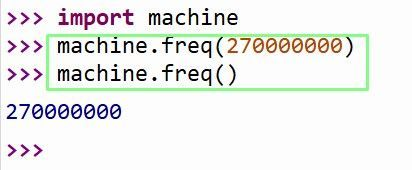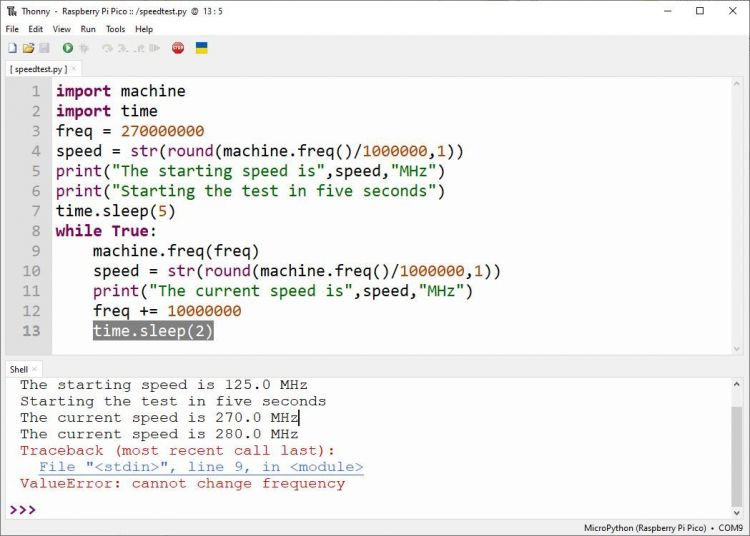# 树莓派 Pico 超频/降频指南## 对树莓派 Pico 超频

```import machine
machine.freq()
````machine.freq(270000000)`

`machine.freq()````import machine
machine.freq(此处填写超频后的 CPU 频率，纯数字，不带单位)
```

## RP2040 超频的上限是多少？

```import machine
import time
```

`freq = 270000000`

`speed = str(round(machine.freq()/1000000,1))`

```print("The starting speed is",speed,"MHz")
```

```print("Starting the test in five seconds")
time.sleep(5)
```

`while True:`

`machine.freq(freq)`

```speed = str(round(machine.freq()/1000000,1))
```

`print("The starting speed is",speed,"MHz")`

`freq += 10000000`

`time.sleep(2)`## 完整代码

```import machine
import time
freq = 270000000
speed = str(round(machine.freq()/1000000,1))
print("The starting speed is",speed,"MHz")
print("Starting the test in five seconds")
time.sleep(5)
while True:
machine.freq(freq)
speed = str(round(machine.freq()/1000000,1))
print("The current speed is",speed,"MHz")
freq += 10000000
time.sleep(2)
```